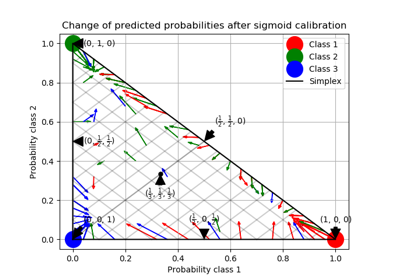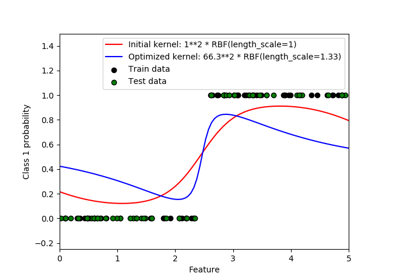# sklearn.metrics.log_loss¶

sklearn.metrics.log_loss(y_true, y_pred, eps=1e-15, normalize=True, sample_weight=None, labels=None)[source]

Log loss, aka logistic loss or cross-entropy loss.

This is the loss function used in (multinomial) logistic regression and extensions of it such as neural networks, defined as the negative log-likelihood of the true labels given a probabilistic classifier’s predictions. The log loss is only defined for two or more labels. For a single sample with true label yt in {0,1} and estimated probability yp that yt = 1, the log loss is

-log P(yt|yp) = -(yt log(yp) + (1 - yt) log(1 - yp))

Read more in the User Guide.

Parameters: y_true : array-like or label indicator matrix Ground truth (correct) labels for n_samples samples. y_pred : array-like of float, shape = (n_samples, n_classes) or (n_samples,) Predicted probabilities, as returned by a classifier’s predict_proba method. If y_pred.shape = (n_samples,) the probabilities provided are assumed to be that of the positive class. The labels in y_pred are assumed to be ordered alphabetically, as done by preprocessing.LabelBinarizer. eps : float Log loss is undefined for p=0 or p=1, so probabilities are clipped to max(eps, min(1 - eps, p)). normalize : bool, optional (default=True) If true, return the mean loss per sample. Otherwise, return the sum of the per-sample losses. sample_weight : array-like of shape = [n_samples], optional Sample weights. labels : array-like, optional (default=None) If not provided, labels will be inferred from y_true. If labels is None and y_pred has shape (n_samples,) the labels are assumed to be binary and are inferred from y_true. .. versionadded:: 0.18 loss : float

Notes

The logarithm used is the natural logarithm (base-e).

References

C.M. Bishop (2006). Pattern Recognition and Machine Learning. Springer, p. 209.

Examples

>>> log_loss(["spam", "ham", "ham", "spam"],
...          [[.1, .9], [.9, .1], [.8, .2], [.35, .65]])
0.21616...


## Examples using sklearn.metrics.log_loss¶Probability Calibration for 3-class classificationProbabilistic predictions with Gaussian process classification (GPC)